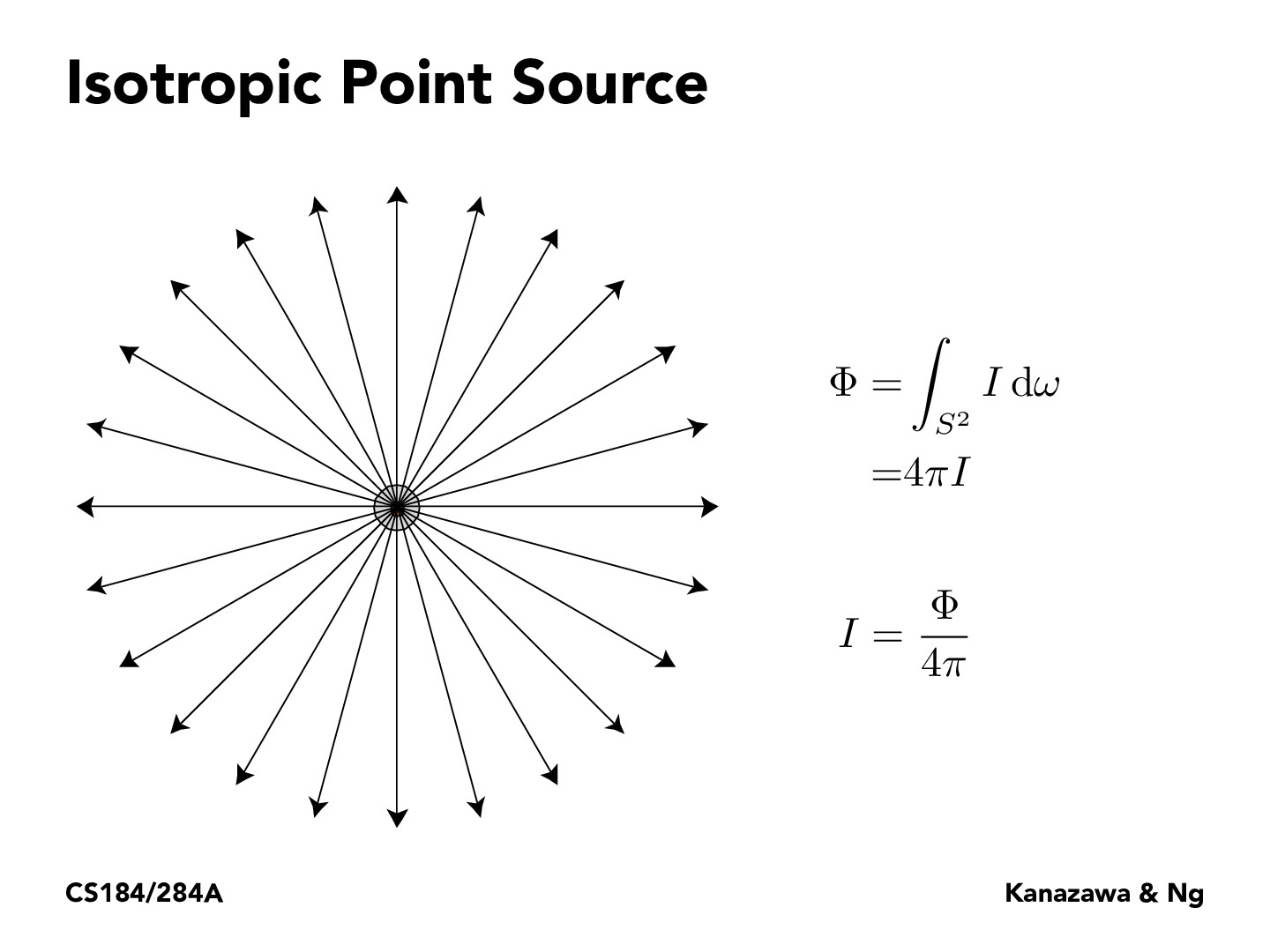Lecture 11: Radiometry & Photometry (18)HJQ2000

Trying to combine the definition together: 4pi is the total amount of solid angles. Luminous intensity I is the power per unit solid angle. Luminous flux is energy per unit time. So intensity = flux / amount of total solid angles. I am a little bit confused on how this formula limited to isotropic point source if the above understanding is correct. Is it because only isotropic point source are sure to have total amount of solid angles of 4pi?tancik

@HJQ2000: It is isotropic because I is constant. In the non isotropic case you would replace I with a solid angle dependent intensity I(w).Leon-Shao

@HJQ2000, I think if isotropic source guaranteed us that in all direction the situation is the same, which will be consistent with the integral result of 4pi. If the point source is not isotropic, you can imaginarily think of a ball surface with "holes" or "thinner" areas that are not covered by those light arrows in the graph.

You must be enrolled in the course to comment